# How to Round Percentages in Excel & Google Sheets

This tutorial will demonstrate how to round percentages in Excel and Google Sheets.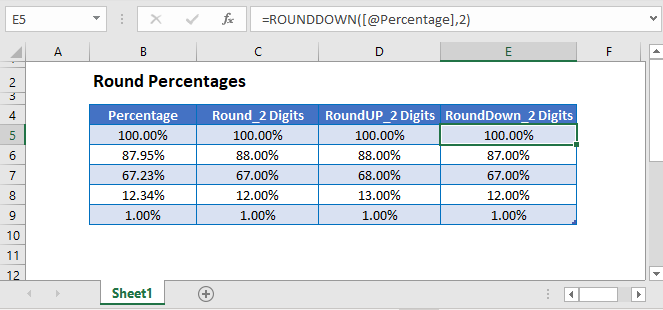## Rounding Percentages

To round numbers in Excel (or Google Sheets), you can use the ROUND Function. When using the ROUND Function you enter the number to round, followed by the number of digits to round the number.

``=ROUND(A2,B2)``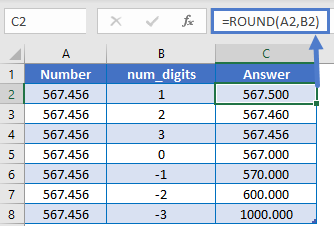This works exactly the same with percentages, however you must remember that a percentages are stored as decimal values. Example: 57.4% is equal to .574: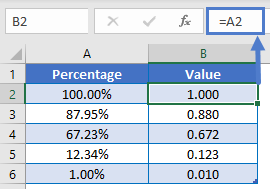So to round percentages we must keep in mind it’s decimal value. To round to the nearest whole percentage use the ROUND Function with num_digits = 2.

``=ROUND(A2,2)``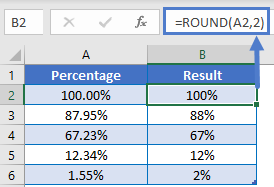To round a percentage to 1 decimal place, we will set num_digits = 3.

``=ROUND(A2,3)``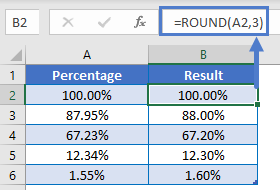### ROUNDUP Function

You can also round up a percentage.

``=ROUNDUP(A2,2)``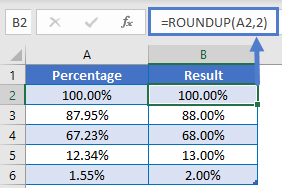### ROUNDDOWN Function

Or round down a percentage.

``=ROUNDDOWN(A2,2)``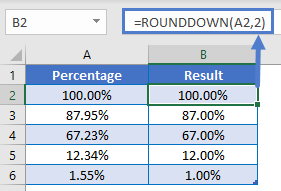## Round Percentages in Google Sheets

All of the above examples work exactly the same in Google Sheets as in Excel.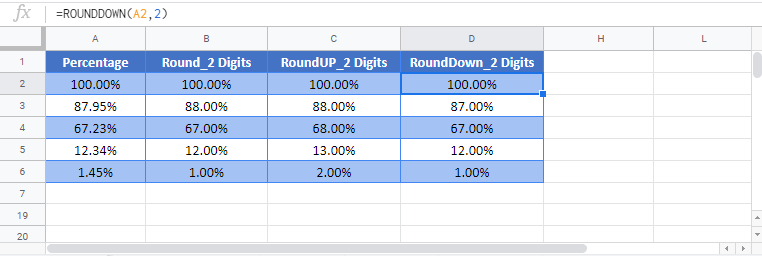### Excel Practice Worksheet

Practice Excel functions and formulas with our 100% free practice worksheets!